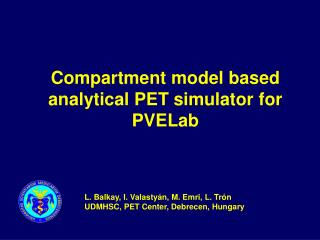DownloadDownload PresentationCompartment model based analytical PET simulator for PVELab

# Compartment model based analytical PET simulator for PVELab

Download Presentation## Compartment model based analytical PET simulator for PVELab

- - - - - - - - - - - - - - - - - - - - - - - - - - - E N D - - - - - - - - - - - - - - - - - - - - - - - - - - -
##### Presentation Transcript

1. Compartment model based analytical PET simulator for PVELab L. Balkay, I. Valastyán,M. Emri, L. Trón UDMHSC, PET Center, Debrecen, Hungary

2. Monte Carlo simulators • (Eidolon PET simulator, SimSET package,…) • tracks each individualg photons from the annihilation to the final absorption or escape • it can take into consideration the PMT characteristics and the whole coincidence signal processing • conceptually precise, versatile, but not fast • Analytical simulators(AS) • (McConnell Brain Imaging Center ,…) • analytically models the most important photon interactions (attenuation, scatter, randoms) • adding poisson noise to sinograms • although less versatile, very fast (~1min/slice) allowing repeated simulation as often as necessary

3. activity distribution forward projection distortion effects on sinograms segmented MRI Correction, reconstruction Analytical PET simulator * * convolving with the PET PSF • Pk = (TkAk+Sk)Nk+Rk • Tk – true counts • Ak – attenuation factors • Sk – scatter counts • Rk – random counts • Nk – normalization factors

4. forward projection distortion effects on sinograms segmented MRI k1 time k2 Correction, reconstruction Option: kinetic model based simulation k1, k2, … maps inputs frames of true activity distortions … tracer kinetic information • model • kinetic const. • blood curve . . .

5. Implementation of kinetic modeling • Generalized matrix representation of dynamic system models X – conc. vector; ,B – matrix, vector of kin. const. The general solution: • Examples:

6. Selectable analytical blood curves:

7. Main steps during the simulation Add the statistical noise (Poisson distribution) Generate the radioactivity distribution and the statistical error of the radioactive decay Spatial blur with PSF Transform to the sinogram space Theoretical activity distribution Simulate the attenuation of the source object Simulate the Compton scattering Simulate the random coincidences Simulate the instrumental and physical effects as Poisson processes Apply the scatter correction Apply the random correction Apply the attenuation correction Correct the distortions of the acquisition Reconstruction,simulated PET image

8. The Matlab GUI

9. GUI to explore the input volume.

10. The GUI for kinetic model definition.

11. The Matlab GUI

12. Validation using the Hoffman slice phantom. Measured and simulated images The simulation time of one dynamic slice took approximately 5 minutes on one 2.8 GHz processor.

13. Simulating C11[FCWAY]accumulation

14. Simulating FDG accumulation T=1.5 min T=2 min T=7 min T=35 min T=60 min T=120 min

15. Simulating F18-L-Dopa accumulation Summed image slice from 40 min to 80 min of simulated dynamic frames

16. Compartment model based analytical PET simulator for PVELab The program can be downloaded from: http:\\pet.dote.hu\pveout